# Heights and Distances – Trigonometry | Class 10 Maths

• Last Updated : 31 Oct, 2020

Heights and Distances are the main application of trigonometry, which is extensively used in the real life. Trigonometry is useful to astronomers, navigators, architects, and surveyors, etc. in solving problems related to heights and distances.

Before going into the problems there are a few terminologies that we used to solve the problem. They are:

• Line of sight: It is the line drawn from the eye of an observer to the point in the object viewed by the observer.
• Angle of impression: The angle between the horizontal and the line of sight joining an observation point to an elevated object is called the angle of elevation.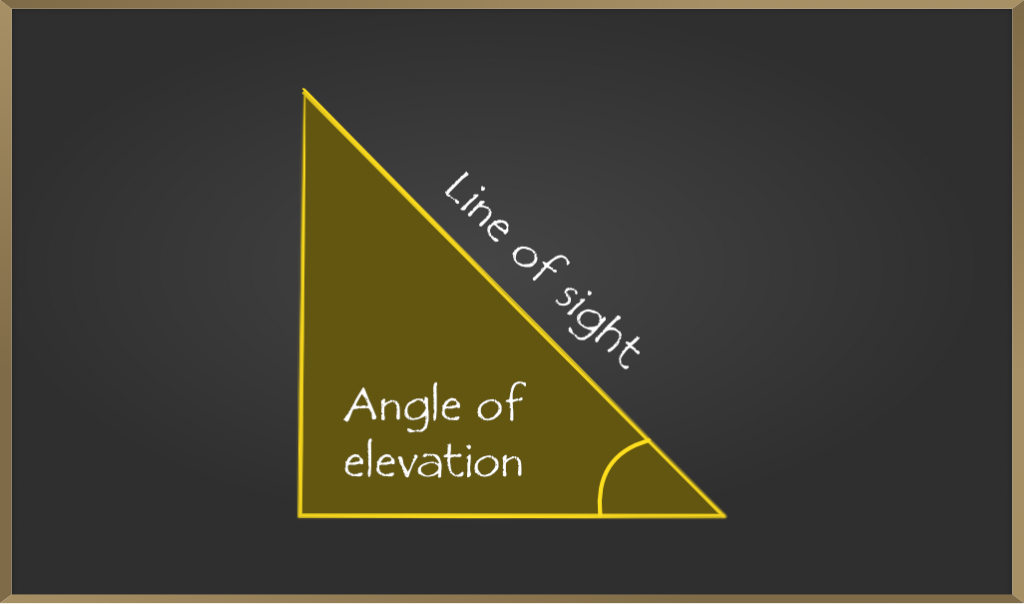• Angle of depression: The angle between the horizontal and the line of sight joining an observation point to an object below the horizontal level is called the angle of depression.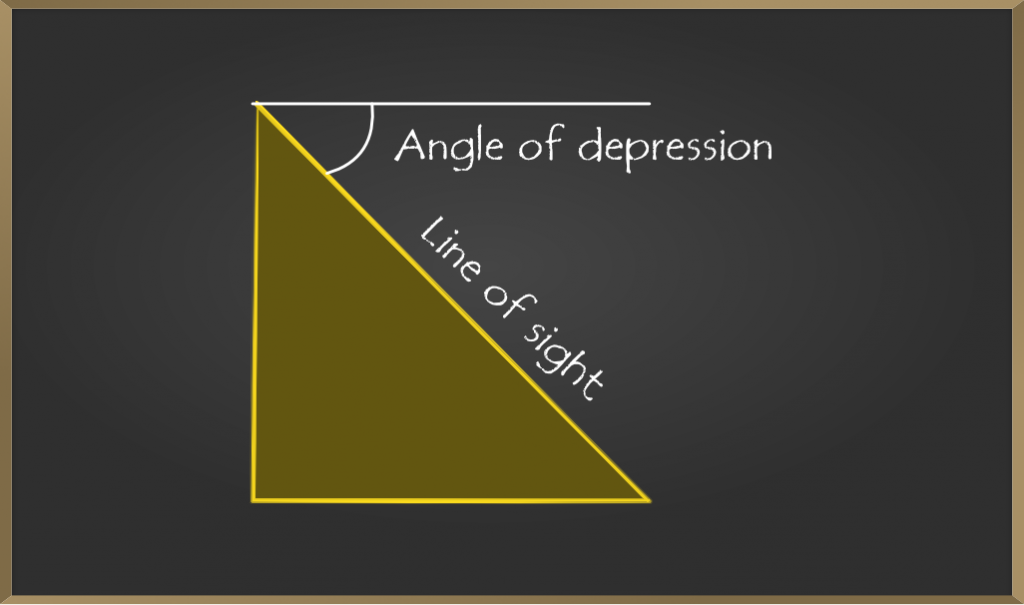## Sample Problems on Height and Distance

Problem 1: If a pole 6 m high casts a shadow 2√3 m long on the ground, find the Sun’s elevation.

Solution: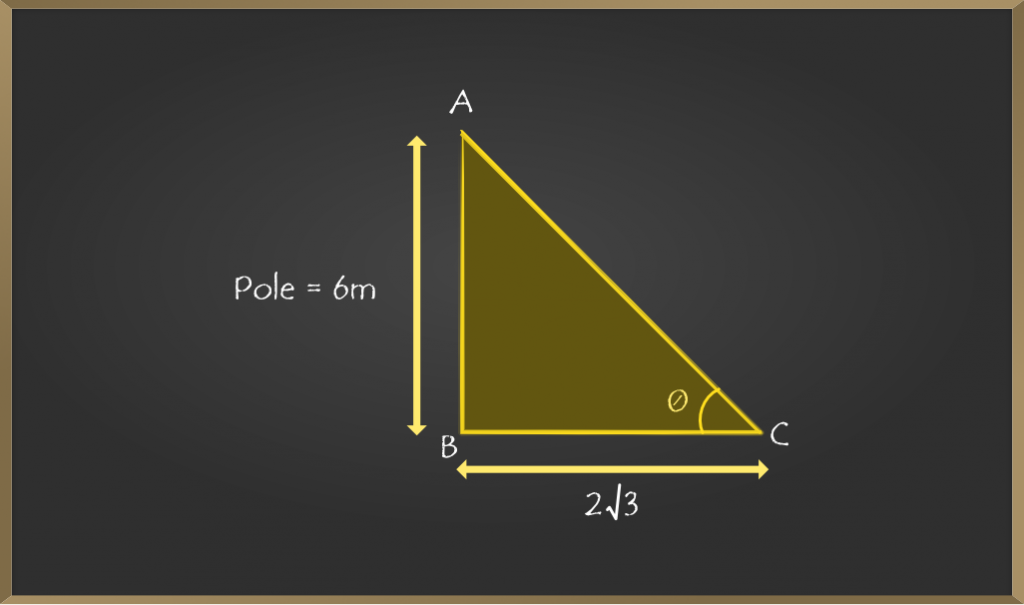Let AB be the pole which is of height 6 m.

Let BC be the shadow of the building 2√3.

Now, in ∆ ABC,

tan θ = AB / BC

=> tan θ = 6 / 2√3

Now, simplifying using rationalization

=> tan θ = (3 / √3)*(√3 / √3)

=> tan θ = 1 / √3

=> θ = tan-1(1 / √3)

Hence, θ = 60o

Therefore, sun’s elevation from the ground is 60o.

Problem 2: An observer 1.5 m tall is 20.5 m away from a tower 22 m high. Determine the angle of elevation of the top of the tower from the eye of the observer.

Solution: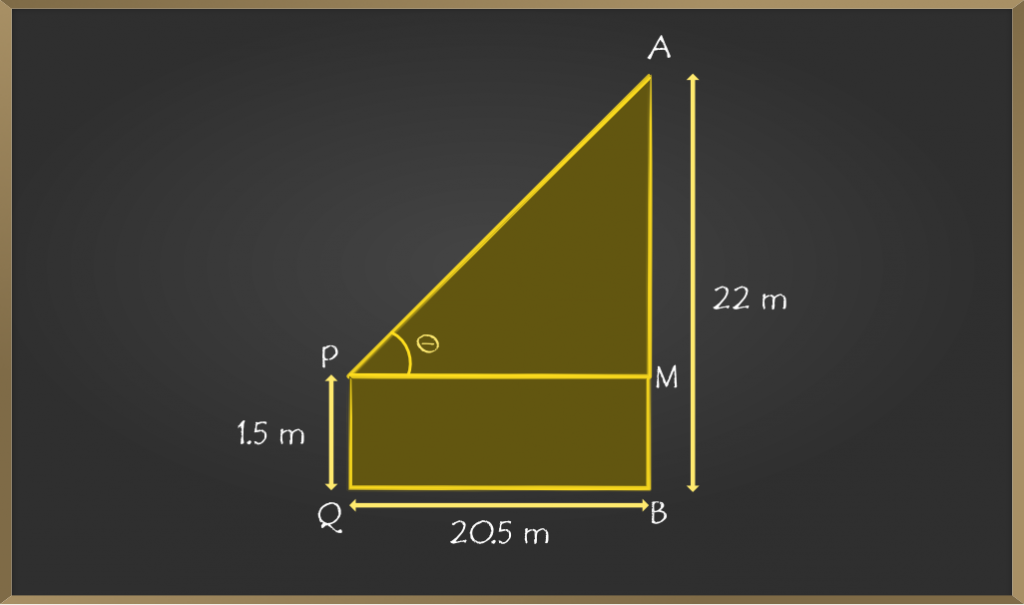Let PQ be the height of the observer of 1.5 m.

Let AB be the height of the tower of 22 m.

And, let QB be the horizontal distance between the observer and the tower

=> PQ = MB = 1.5 m

=> AM = AB – MB

=> AM = 22 – 1.5 = 20.5

Now, in ∆APM,

=> tan θ = AM / PM

=> tan θ = 20.5 / 20.5

=> tan θ = 1

=> θ = tan-1(1 )

Hence, θ = 45o

Therefore, the angle of elevation of the top of the tower from the eye of the observer is 45o

Problem 3: An airplane is flying h meters above the ground. At a particular instant, the angle of elevation of the plane from the eyes of a boy sitting on the ground is 60°. After some time, the angle of elevation changed to 30°. Find the distance covered by the plane during that time assuming it traveled in a straight line.

Solution: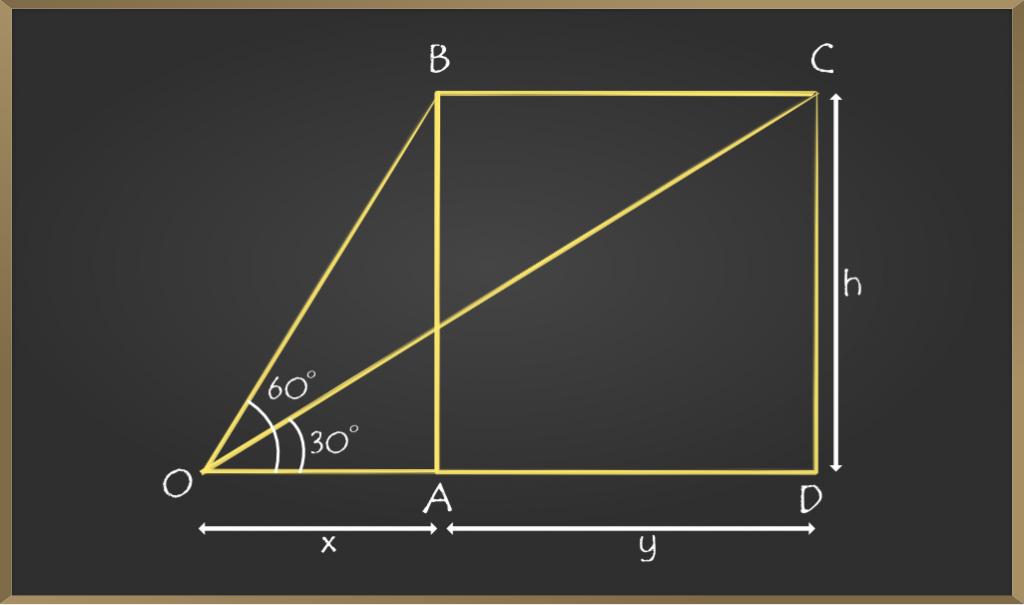Let x be the horizontal distance between the observer and plane at the first instant.

Let y be the horizontal distance between the observer and plane at the second instant.

And, BA = CD = h

In ∆OAB,

=> tan 60° = AB / OA

=> √3 = h / x

=> x = h / √3

In ∆ OCD,

=> tan 30° = CD / OD

=> 1/√3 = h / (x+y)

=> x + y = √3h

Distance travelled by plane = AD = y

=> (x + y) − x = √3h − h / √3

=> y = (2 / √3)h

So, if the airplane is flying h meters above the ground, it would travel for (2/√3) h meters as the angle of elevation changes from 60° to 30°.

Problem 4: From the top of the tower 30 m height a man is observing the base of a tree at an angle of depression measuring 30 degrees. Find the distance between the tree and the tower.

Solution: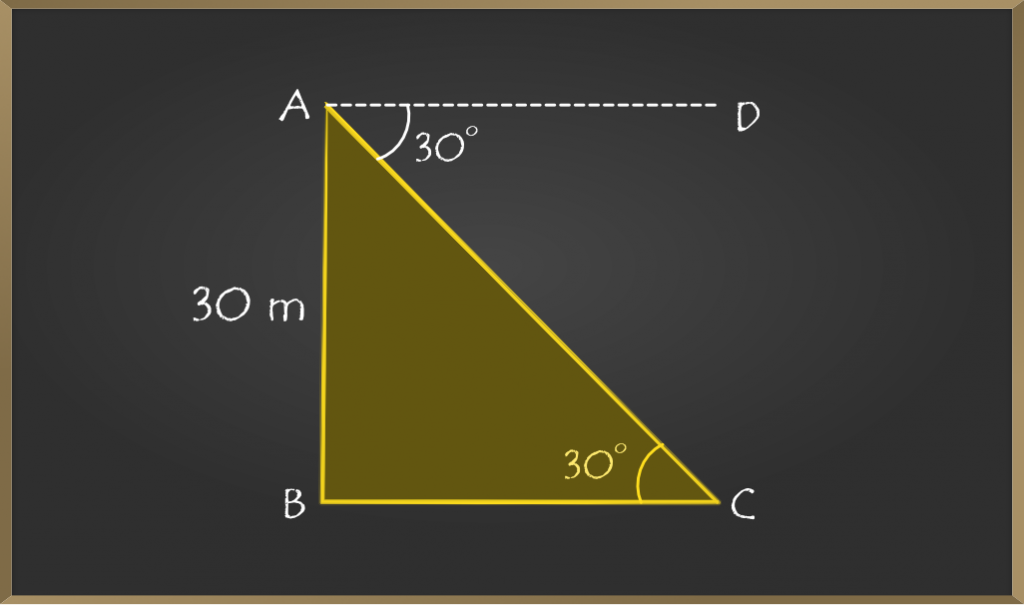In the above diagram AB represents the height of the tower, BC represents the distance between the foot of the tower and the foot of the tree.

Now we need to find the distance between the foot of the tower and the foot of the tree (BC). For that as angle of depression is given so by vertically opposite angle property of triangle ∠CAD = ∠BCA

In ∆BCA,

=> tan θ = Opposite side / Adjacent side

=> tan 30° = AB / BC

=> 1/√3 = 30 / BC

=> BC = 30√3

=> BC = 30 (1.732)      [Approximately]

=> BC = 51.96 m

So, the distance between the tree and the tower is 51.96 m.

Problem 5: From the top of a building 30 m high, the top and bottom of a tower are observed to have angles of depression 30° and 45° respectively. Find the height of the tower.

Solution: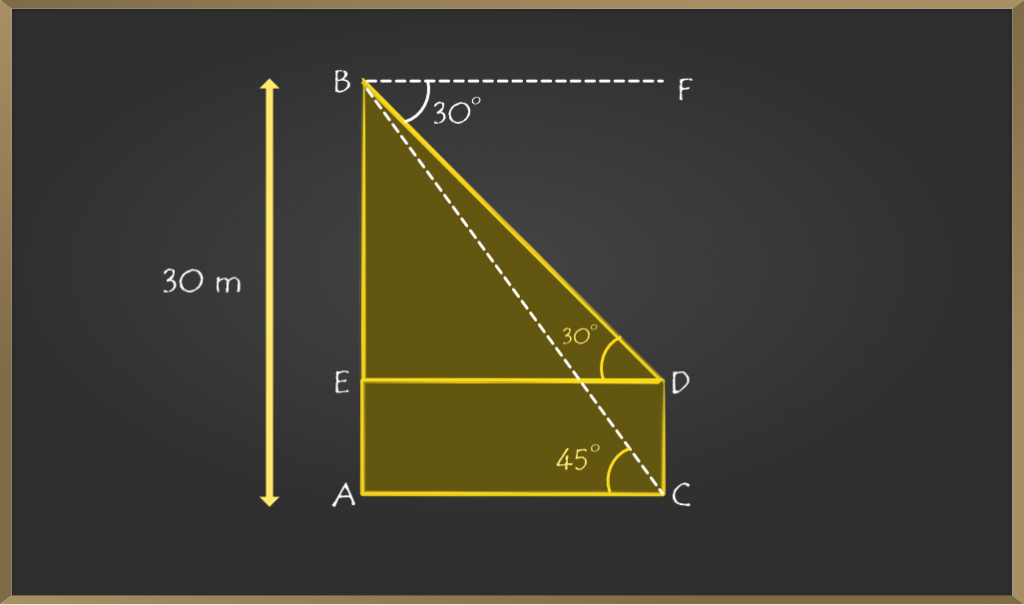Let AB be the building and CD be the tower.

The angle of depressions is given 30° and 45° to the top and bottom of the tower. So by vertically opposite triangle property ∠FBD = ∠EDB and ∠FBC = ∠ACB.

Now, AB = 30 m. Let DC = x.

Draw DE perpendicular AB. Then AE = CD = x.

Therefore  BE = (30 – x) m.

In ∆ACB,

=> cot θ = Adjacent side / Opposite side

=> cot θ = AC / AB

=> cot 45° = AC / 30

=> AC = 30              [cot 45° = 1]

So, DE = AC = 30 m

In ∆EDB,

=> tan θ = Opposite side / Adjacent side

=> tan 30° = BE / DE

=> 1/√3 = BE / 30

=> BE = 30 / √3

=> CD = AE = AB – BE = 30 – (30 / √3)

=> 30[1 – (1 / √3) ] m

Height of the tower is 30[1 – (1 / √3) ] m

My Personal Notes arrow_drop_up